Click here to check the course website.

Supervision work is due by 7pm (19:00) on the day before the supervision. Partial work is perfectly fine as long as it's clear that you made an effort and it is visible from your work where you got stuck.

Feel free to attach any questions to your work.

Supervision questions based on the question set by the lecturer.

## Revision questions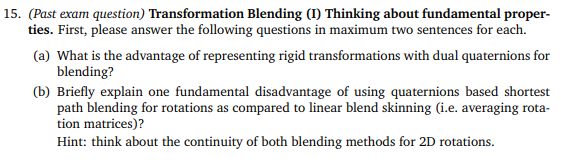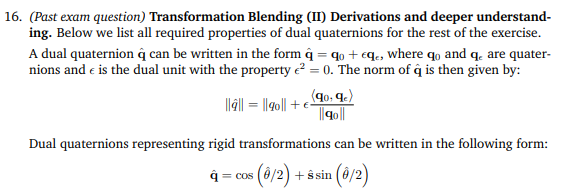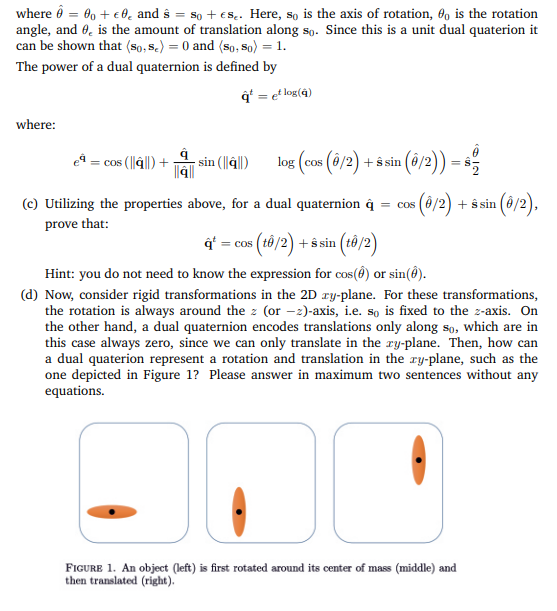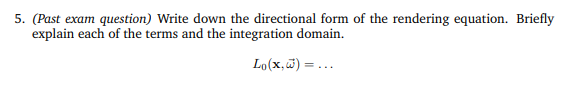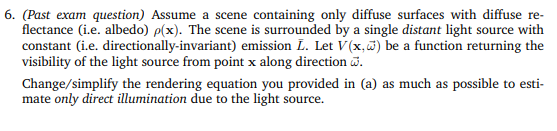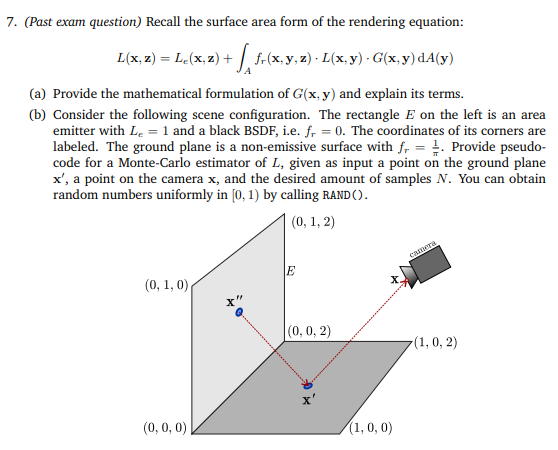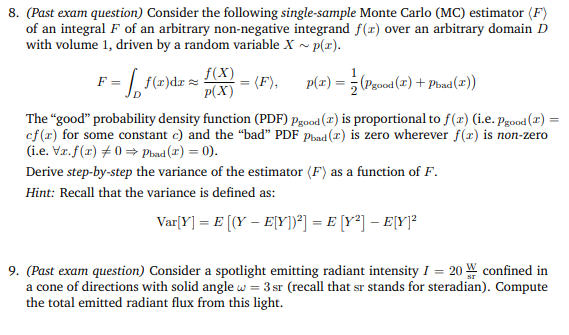## Supervision 1

### Warmup questions

1. Name four different ways to represent geometry
2. What is a manifold and how does it differ from a manifold with boundaries ?
3. What is the parametric representation of a circle in 2D?
4. What is the implicit curve representation of a circle in 2D?
5. How do you compute the surface normal n for a parametric surface?
6. What data structure is used to represent triangle meshes? That is: how is the data that describes a triangle mesh stored? Which parts of this data structure pertain to the topology and which to the geometry of a surface?

### Longer questions

1. (Briefly) compare and contrast the different ways to represent geometry. Focus on the advantages and disadvantages of each approach. (This is not an essay question; a table/list or some equivalent works probably best).
2. Which geometry representations work well with texture mapping?
3. What geometric shape does the following planar curve describe?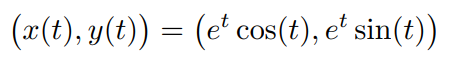4. Below you find the parametrisation of a torus. Compute the surface normal n for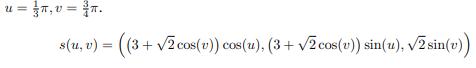5. The following implicit equation describes an ellipsoid in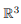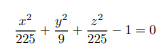1. Find a parametric equation that describes the same ellipsis. Hint: start off with the parametric equation of a sphere and then modify
2. Prove that P = (2, 2, −11) lies on the ellipsoid’s surface.
3. Determine the surface normal n at point P .
6. If we define a curve in R 3 with the implicit function f(x, y) = x 2 y 2 , what problem could there arise when computing the surface normal?

7.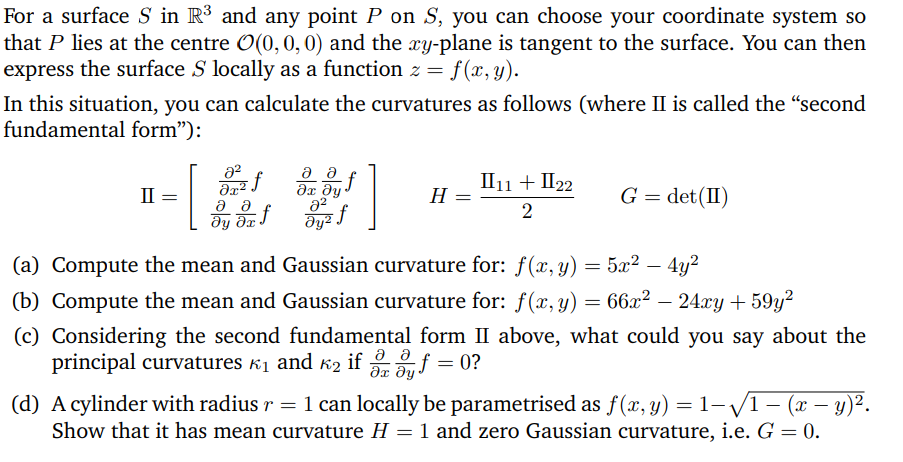8. Compute the Laplacian of the given functions f(x) (assume that everything except for x is constant).
1.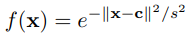2.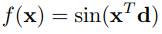9.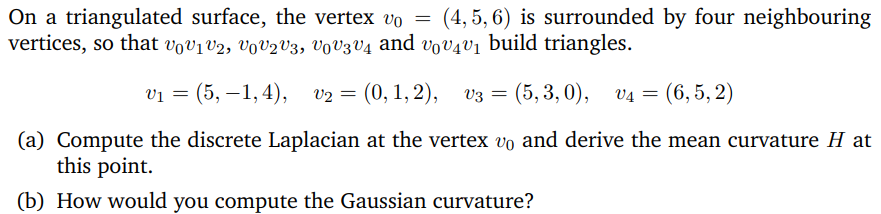10.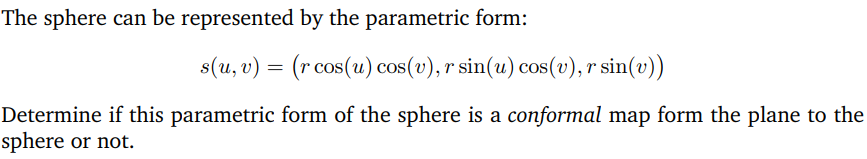## Supervision 2

### Warmup questions

1. What is rigging? Briefly describe what kind of structure and data are added to the geometry/surface model.
2. What is the difference between active and passive optical scanning systems and what are the advantages of passive systems?
3. Briefly describe the steps of the iterative closest point algorithm.
4. What is the advantage of representing rigid transformations with dual quaternions for blending?
5. Briefly explain one fundamental disadvantage of using quaternions based shortest path blending for rotations as compared to linear blend skinning (i.e. averaging rotation matrices).
6. What is the BRDF?
7. What is the rendering equation?
8. How is path tracing an example of a Monte Carlo Algorithm?